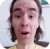FreeKB - Print number numbers and count lines in a file in Perl (\$.)
Print number numbers and count lines in a file in Perl (\$.)Let's say foo.txt contains 5 lines of text.

``````Line one
Line two
Line three
Line four
Line five``````

\$. is the default Perl variable that captures the number of lines in a file.

``````open(FH, "<", "foo.txt");

while (my \$line = <FH>) {
print "\$. \$line";
}

close FH;``````

The prior markup used \$. to print each line number and \$line to print each line, which would produce the following output.

``````1 Line one
2 Line two
3 Line three
4 Line four
5 Line five``````

This will count the total number of lines in the file.

``````open(FH, "<", "foo.txt");

my \$count = 0;

while (<FH>) {
\$count = \$.;
}

close FH;

print "\$count\n";``````

To count the number of lines in a file that contain the text "Hello World".

``````my \$file = "/path/to/file.txt";
open(FH, "<", \$file) or die "cannot open \$file \$! \n";

my \$count = 0;

while (<FH>) {
\$count++ if /Hello World/;
}

close FH;

print "\$count\n";``````

We will never share your name or email with anyone. Enter your email if you would like to be notified when we respond to your comment.

Please enter c893c in the box below so that we can be sure you are a human.